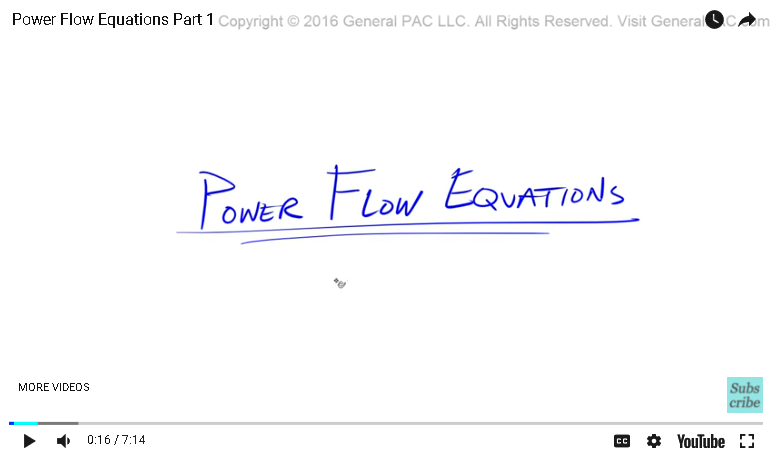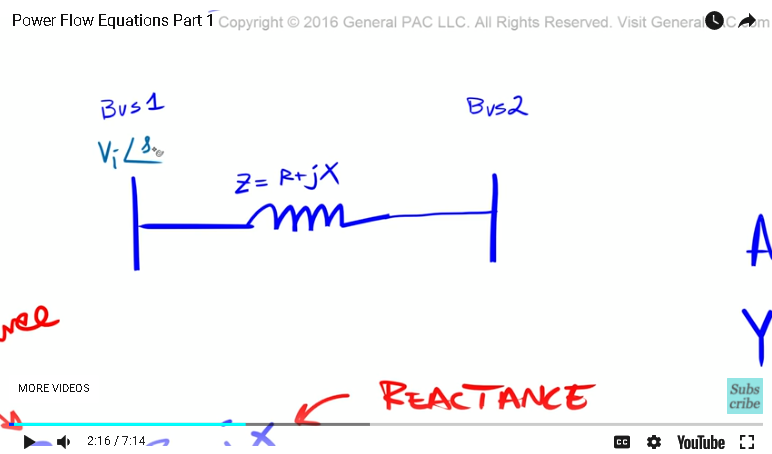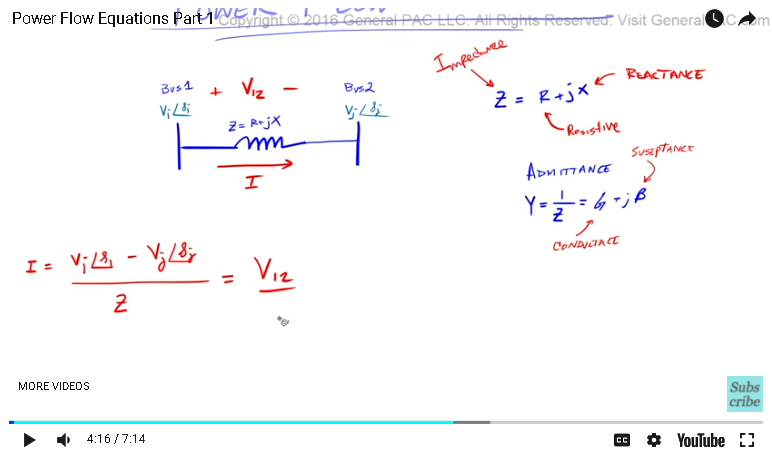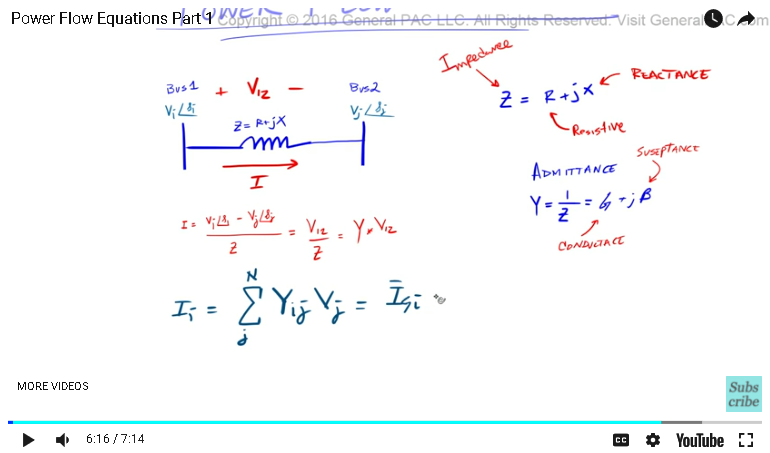This video was brought to you by GeneralPAC.com, making power systems Intuitive, Open and Free for Everyone, Everywhere. Consider subscribing and supporting through patreon.com/GeneralPAC. This is a mechanism for you to support us financially so we can continue making high quality power system video tutorials. Our corporate sponsor for this topic is AllumiaX.com from Seattle, Washington. Contact them for industrial and commercial power system studies.

Power flow equations Part 1

In this video tutorial, we'll be talking about the very common power flow equations that are often found in textbooks and classrooms, but we're gonna be looking at them from an intuitive perspective.This is part one. When we have a conductor that spans from bus one to bus two, this conductor, or the transmission line, has some impedance. To model this impedance, we have to have resistive component and a reactive component. And that, essentially equals: z=r+jx. The r, is the resistive component of the transmission line. The x, is the reactive component, or the reactants. And then z, is called impedance. The impedance is the resistive plus reactants.

Another way to describe impedance is a term called admittance. Admittance is essentially one over the impedance. That's the admittance. If we think about it, impedance is the amount of impedance that the current will receive if it goes, or if it travels from bus one to bus two. Right? Impedance. Which, kind of, makes sense in the English language.

And then, admittance is the reciprocal of impedance or one over the impedance. So, admittance is how easy the current is allowed to travel from bus one to bus two through this transmission line. So, admittance is one over the impedance and that also equals g+jb. Where g, is called the conductance and b is called the susceptance. Okay.

Next, let's talk about the voltages. Let's zoom in here. The voltage on bus one, we'll call it vI. Right? Don't mind the subscript for now, it's very important for later. So vI, at some angle, tilda, I. And so the voltage on bus two, we'll call that voltage vJ. K? And then, the angle of that voltage is tilda J. So we have two different voltages. The voltage on bus one and the voltage on bus two.Let me make these a little bit smaller. Now, lets just talk about these subscripts. These subscripts are important because the power system is obviously not a two bus system. The power system is very big, very large, and so we need these subscripts to define each individual buses. If we want to determine the current that's flowing from bus one to bus two, how would we do it?

The simplest way to think about it is, current is equal to the voltage drop between bus one and bus two. So we'll say that we have the voltage of bus one, which is vI at some angle I minus the voltage one bus two, which is vJ, right, at angle J. And then, we divide that by the impedance, which makes perfect sense. Right? Makes total sense.

Now let's move that over here. The other way to describe this current is, if we knew this impedance, if we knew z, and if we already knew the voltage. So the voltage between bus one and bus two. Let's call this v12. If we already knew what the voltage is, which we already know what these two terms are and we know what the impedance of the transmission line is, then the current is equal to the voltage between bus one and bus two, which is this voltage here, which is again, this voltage there. You divide that by the impedance of the line, which is what we said over here. Right? Makes sense. Okay.Now, let's try to use this admittance. We said the admittance was y is equal to 1 over z. Okay? If y is equal to 1 over z, what if we just took y, the admittance of this transmission line, and then we just multiplied that by v12. That still makes sense because all we're doing is, instead of calling this impedance, we're gonna call it admittance and look, our equation is so much more simpler. Right? It's just admittance times the voltage draw between bus one and bus two.

Let's make this smaller. Now if we were to do this particular equation with the respect to a multi-bus system, how would we do it? Meaning, let's add some scripts to this equation so that we can define the current to all of the buses in our system. We would do that by this method. This method is very much documented in a lot of textbooks.

We're gonna say I, which is basically akin to this current here, or akin to the current that's flowing to the transmission line. But we're gonna say the current in the I bus, right, that is equal to the sum of the currents, and we're gonna call this j, to the end number of buses in the system. So the summation of Yij, times that by Vj. This is the way to define current in all of the buses in our system and that is equal to, essentially, the current generated at a bus. K? The current generated at a bus minus the load current on that bus.We'll cover this later. It's essentially, this is the equation that we're very much interested in. Okay so now that we've talked about current, our next step is to talk about actual power, which is defined by S. Right? The apparent power, but before we talk about this, if you haven't subscribed already, please go ahead and do so by clicking on the bottom right corner of the screen. Click on the subscribe button and subscribe to this channel.

Now if you have any questions, click on the link that's shown on the bottom of this video and it'll take you to a forum. The forum is hosted by generalpac.com, and it serves the purpose of asking questions and the community responding to those questions. Go to the forum at generalpac.com and ask away. Thank you.

#### Greetings from the GeneralPAC Team!

We make high-quality Power Systems Video Tutorials on complex topics that are free and open to everyone!  Thank you so much for supporting us through Patreon so we can continue doing good and valuable work.

What is Patreon and why do we use it?

Patreon is a fantastic portal that allows our fans and community to make monthly contribution (like Netflix subscription) so we can continue creating high-quality power systems video tutorials. In return, you get access to incredible perks like voting on future topics, getting your questions answered, access to VIP Q/A webinars with the creators of GeneralPAC, and much more! We THANK YOU for supporting us

Why do we need your support?

An incredible amount of time and effort is needed to develop high-quality video tutorials. Each video (Part 1 for example) takes approximately 10 hours to complete which includes learning the concept ourselves, brainstorming creative ways to teach and explain the concepts, writing the script, audio recording, video recording, and editing. It's no wonder why Hundreds-of-Thousands of people have watched, liked, subscribed, and left positive comments on Youtube channel. Your support truly makes all the difference.